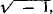# imaginary number

(redirected from I number)
Also found in: Dictionary, Thesaurus.

## imaginary number:

see numbernumber,
entity describing the magnitude or position of a mathematical object or extensions of these concepts. The Natural Numbers

Cardinal numbers describe the size of a collection of objects; two such collections have the same (cardinal) number of objects if their
.

## Imaginary Number

a number of the form x + iy, where i =x and y are real numbers and y ≠ 0; that is, a complex number that is not real. Imaginary numbers of the form iy are called pure imaginary; sometimes only the latter are referred to as imaginary numbers. The term “imaginary number” appeared after such numbers had already entered general use although their real meaning had not been ascertained.

## imaginary number

[ə′maj·ə‚ner·ē ′nəm·bər]
(mathematics)
A complex number of the form a + bi, with b not equal to zero, where a and b are real numbers, and i = √(-1); some mathematicians require also that a = 0. Also known as imaginary quantity.
Site: Follow: Share:
Open / Close